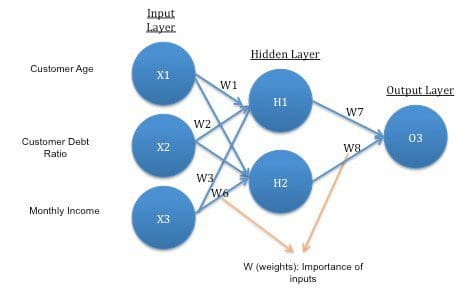#Introduction to Neural Networks, Advantages and Applications

Artificial Neural Network (ANN) algorithm mimic the human brain to process information. Here we explain how human brain and ANN works.

By Jahnavi Mahanta.

Artificial Neural Network (ANN) uses the processing of the brain as a basis to develop algorithms that can be used to model complex patterns and prediction problems.

Lets begin by first understanding how our brain processes information:

In our brain, there are billions of cells called neurons, which processes information in the form of electric signals. External information/stimuli is received by the dendrites of the neuron, proccessed in the neuron cell body, converted to an output and passed through the Axon to the next neuron. The next neuron can choose to either accept it or reject it depending on the strength of the signal.Now, lets try to understand how a ANN works:Here, w1, w2, w3 gives the strength of the input signals.

As you can see from the above, an ANN is a very simplistic representation of a how a brain neuron works.

To make things clearer, lets understand ANN using a simple example: A bank wants to assess whether to approve a loan application to a customer, so, it wants to predict whether a customer is likely to default on the loan. It has data like below:So, we have to predict Column X. A prediction closer to 1 indicates that the customer has more chances to default.

Lets try to create an Artificial Neural Network architecture loosely based on the structure of a neuron using this example:In general, a simple ANN architecture for the above example could be:Key Points related to the architecture:

1. The network architecture has an input layer, hidden layer (there can be more than 1) and the output layer. It is also called MLP (Multi Layer Perceptron) because of the multiple layers.
2. The hidden layer can be seen as a “distillation layer” that distills some of the important patterns from the inputs and passes it onto the next layer to see. It makes the network faster and efficient by identifying only the important information from the inputs leaving out the redundant information
3. The activation function serves two notable purposes:
• It captures non-linear relationship between the inputs
• It helps convert the input into a more useful output.

In the above example, the activation function used is sigmoid:
O1 = 1 / 1+e-F
Where F = W1*X1 + W2*X2 + W3*X3

Sigmoid activation function creates an output with values between 0 and 1. There can be other activation functions like Tanh, softmax and RELU.

1. Similarly, the hidden layer leads to the final prediction at the output layer:

O3 = 1 / 1+e-F 1

Where F 1= W7*H1 + W8*H2

Here, the output value (O3) is between 0 and 1. A value closer to 1 (e.g. 0.75) indicates that there is a higher indication of customer defaulting.